# Conic sections questions and answers

Recent questions in Conic sectionsossidianaZ 2021-08-10 Answered

### Find the equation of the graph for each conic in general form. Identify the conic, the center, the vertex, the co-vertex, the focus (foci), major axis, minor axis, $$\displaystyle{a}^{{2}}$$, $$\displaystyle{b}^{{2}}$$, and $$\displaystyle{c}^{{2}}$$. For hyperbola,find the asymtotes.Sketch the graph $$\displaystyle{2}{\left({y}+{2}\right)}^{{2}}+{4}{\left({x}-{4}\right)}^{{2}}={8}$$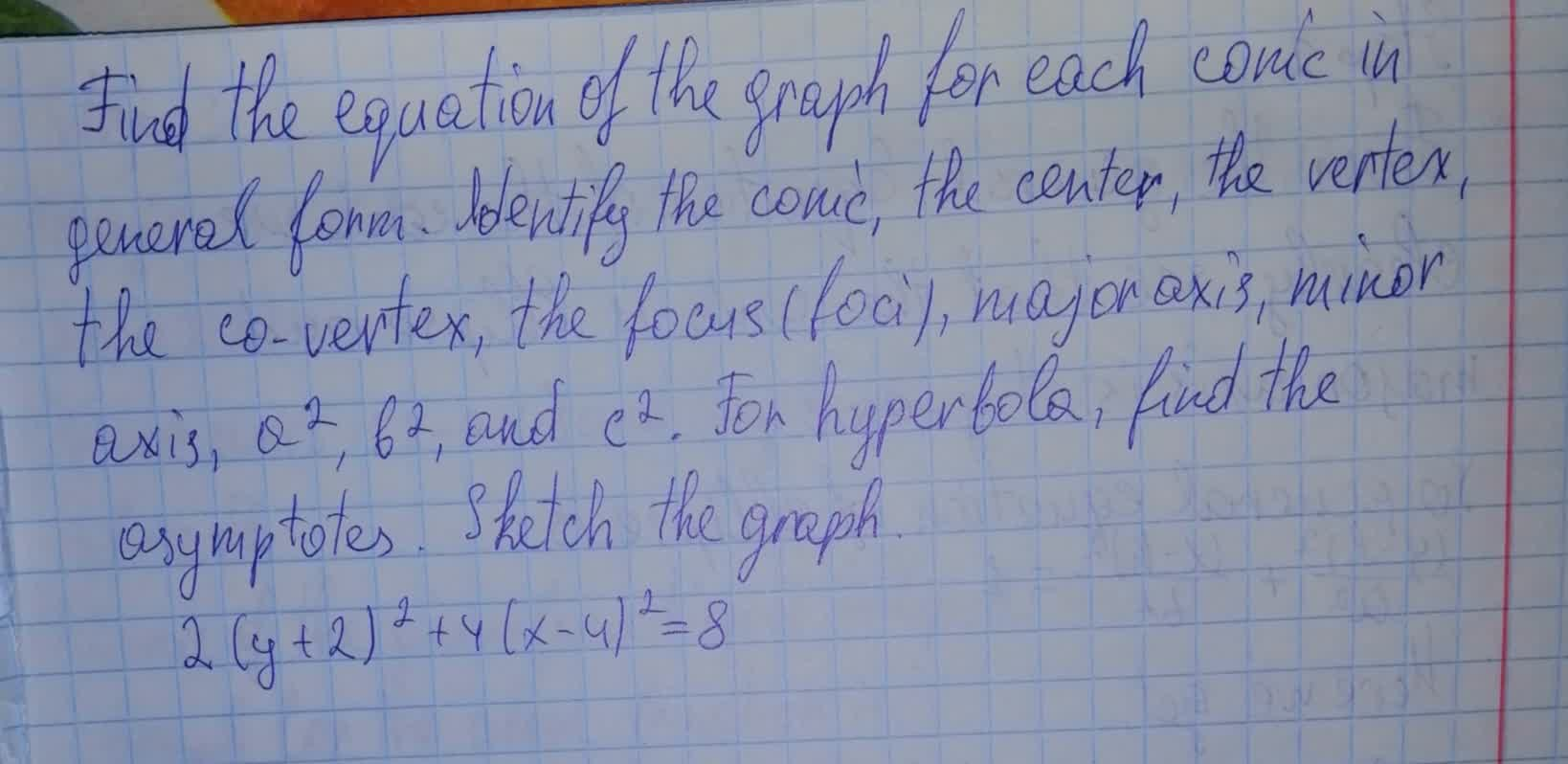nagasenaz 2021-08-10 Answered

### Find the equation of the graph for each conic in general form. Identify the conic, the center, the vertex, the co-vertex, the focus (foci), major axis, minor axis, $$\displaystyle{a}^{{2}}$$, $$\displaystyle{b}^{{2}}$$, and $$\displaystyle{c}^{{2}}$$. For hyperbola,find the asymtotes.Sketch the graph $$\displaystyle{x}+{2}{y}^{{2}}={4}$$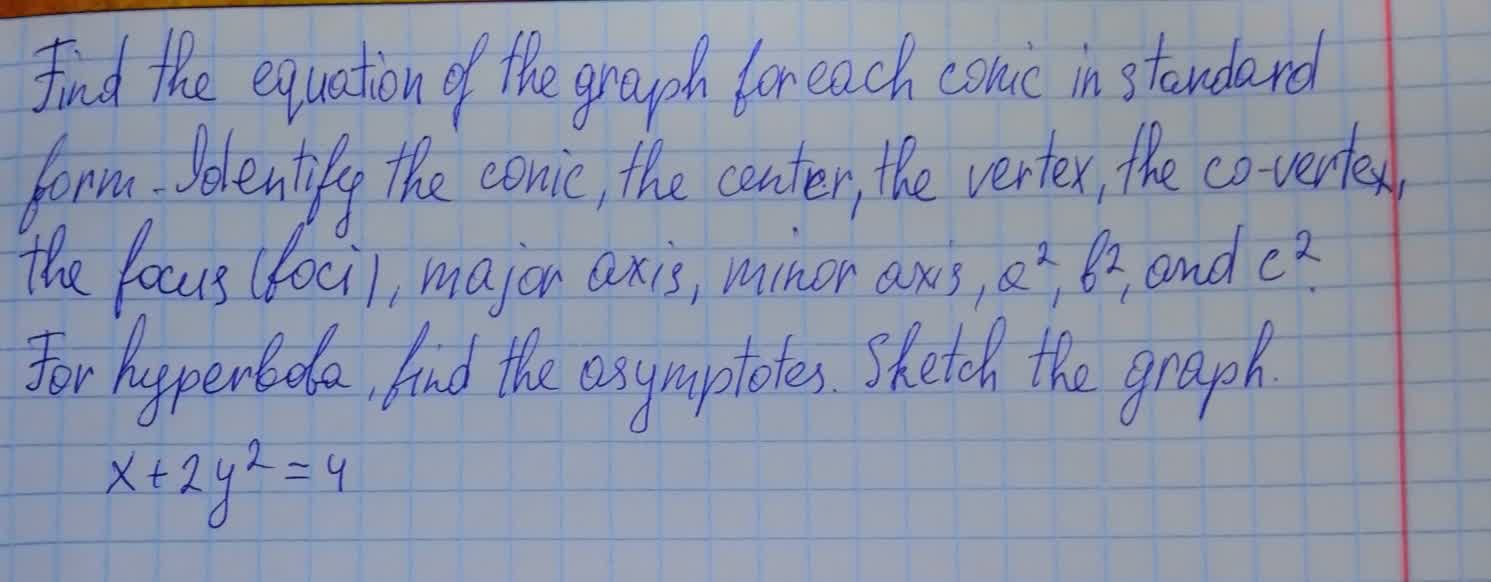Efan Halliday 2021-08-10 Answered

### Perform a rotation of axes to eliminate the xy-term, and sketch the graph of the “degenerate” conic.$$\displaystyle{x}^{{2}}+{2}{x}{y}+{y}^{{2}}-{1}={0}$$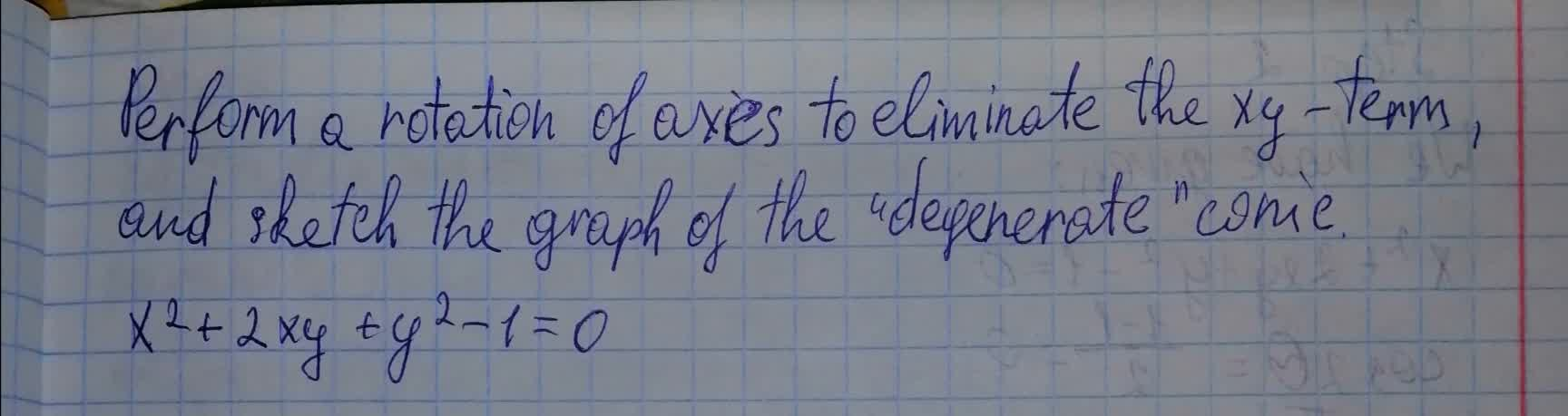kuCAu 2021-08-09 Answered

### Use the information provided to write the general conic form equation of each ellipse. $$\displaystyle{\frac{{{x}^{{2}}}}{{{36}}}}+{\frac{{{y}^{{2}}}}{{{81}}}}={1}$$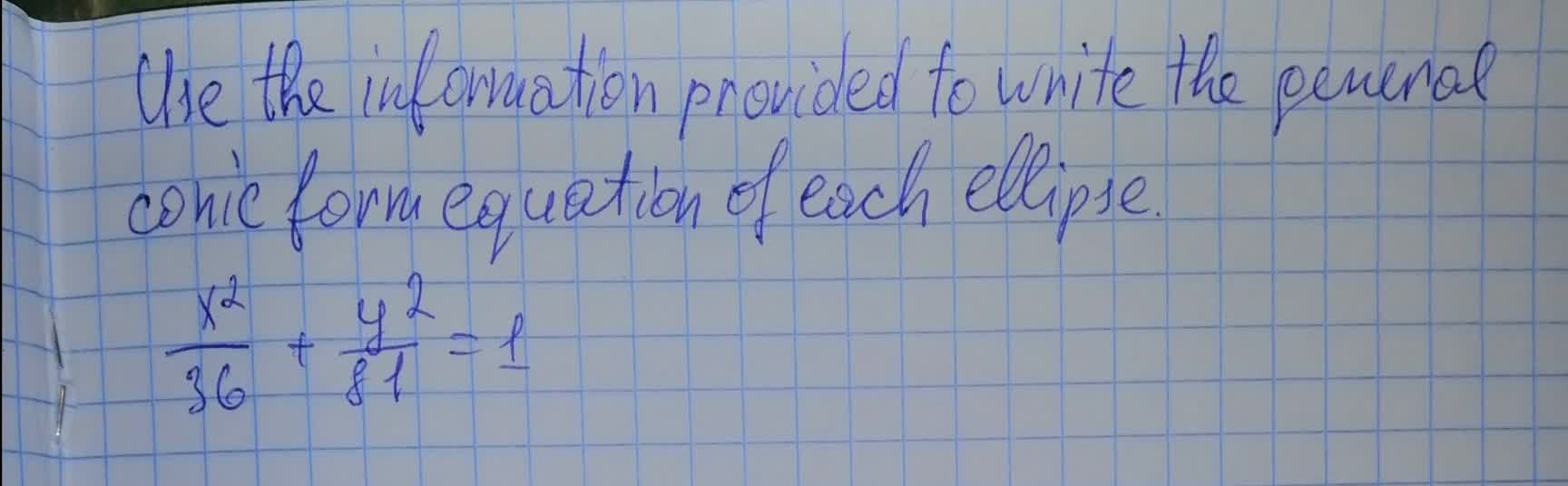bobbie71G 2021-08-09 Answered

### Show that the equation represents a conic section. Sketch the conic section, and indicate all pertinent information (such as foci, directrix, asymptotes, and so on). $$\displaystyle{\left({a}\right)}{x}^{{2}}–{2}{x}–{4}{y}^{{2}}–{12}{y}=-{8}{\left({b}\right)}{2}{x}^{{2}}+{4}{x}-{5}{y}+{7}={0}{\left({c}\right)}{8}{a}^{{2}}+{8}{x}+{2}{y}^{{2}}–{20}{y}={12}$$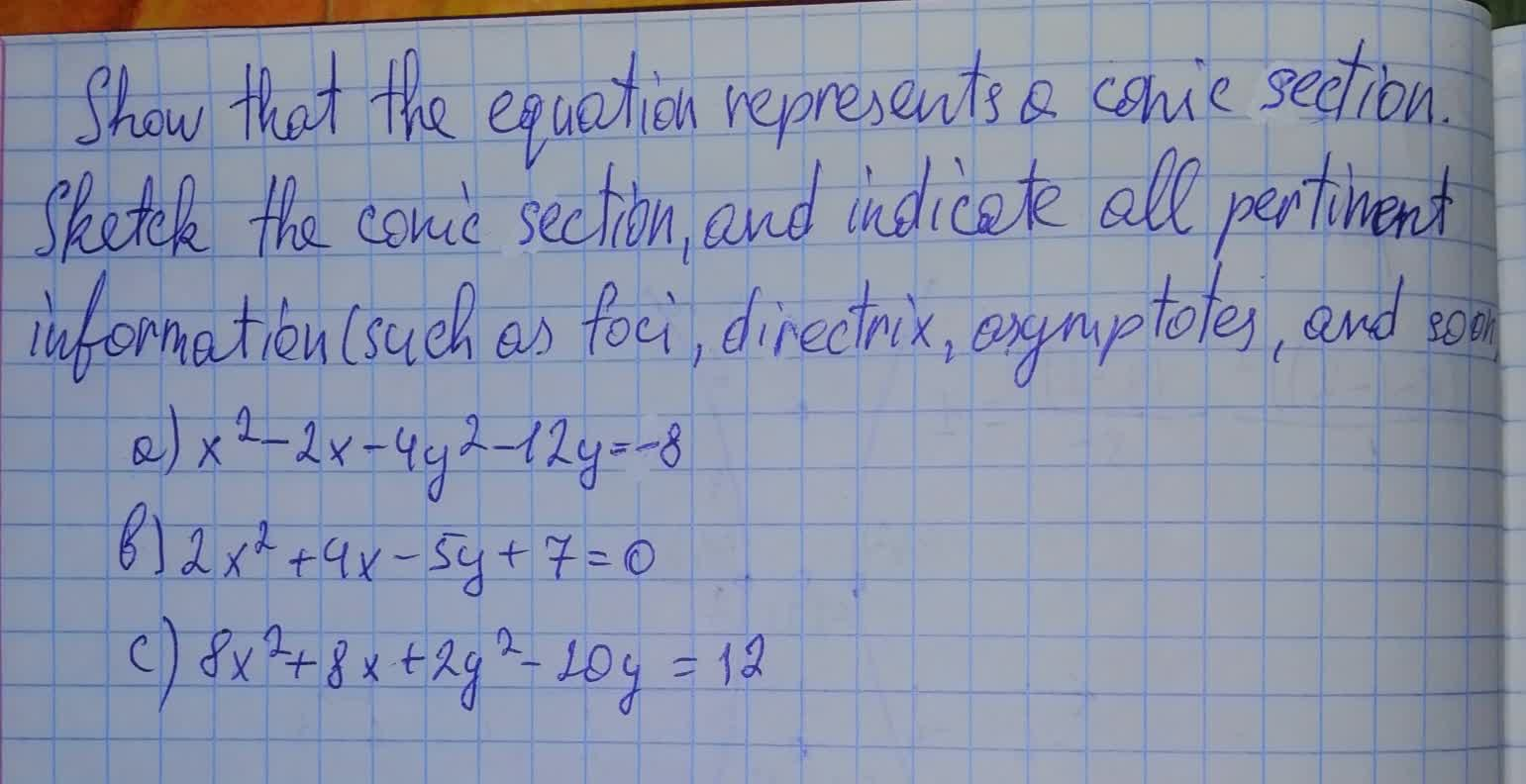preprekomW 2021-08-09 Answered

### Find the equation of the graph for each conic in standard form. Identify the conic, the center, the vertex, the co-vertex, the focus (foci), major axis, minor axis, $$\displaystyle{a}^{{2}},{b}^{{2}}$$, and $$\displaystyle{c}^{{2}}$$?. For hyperbola, find the asymptotes. Sketch the graph. $$\displaystyle{y}^{{2}}+{4}{y}-{2}{x}+{6}={0}$$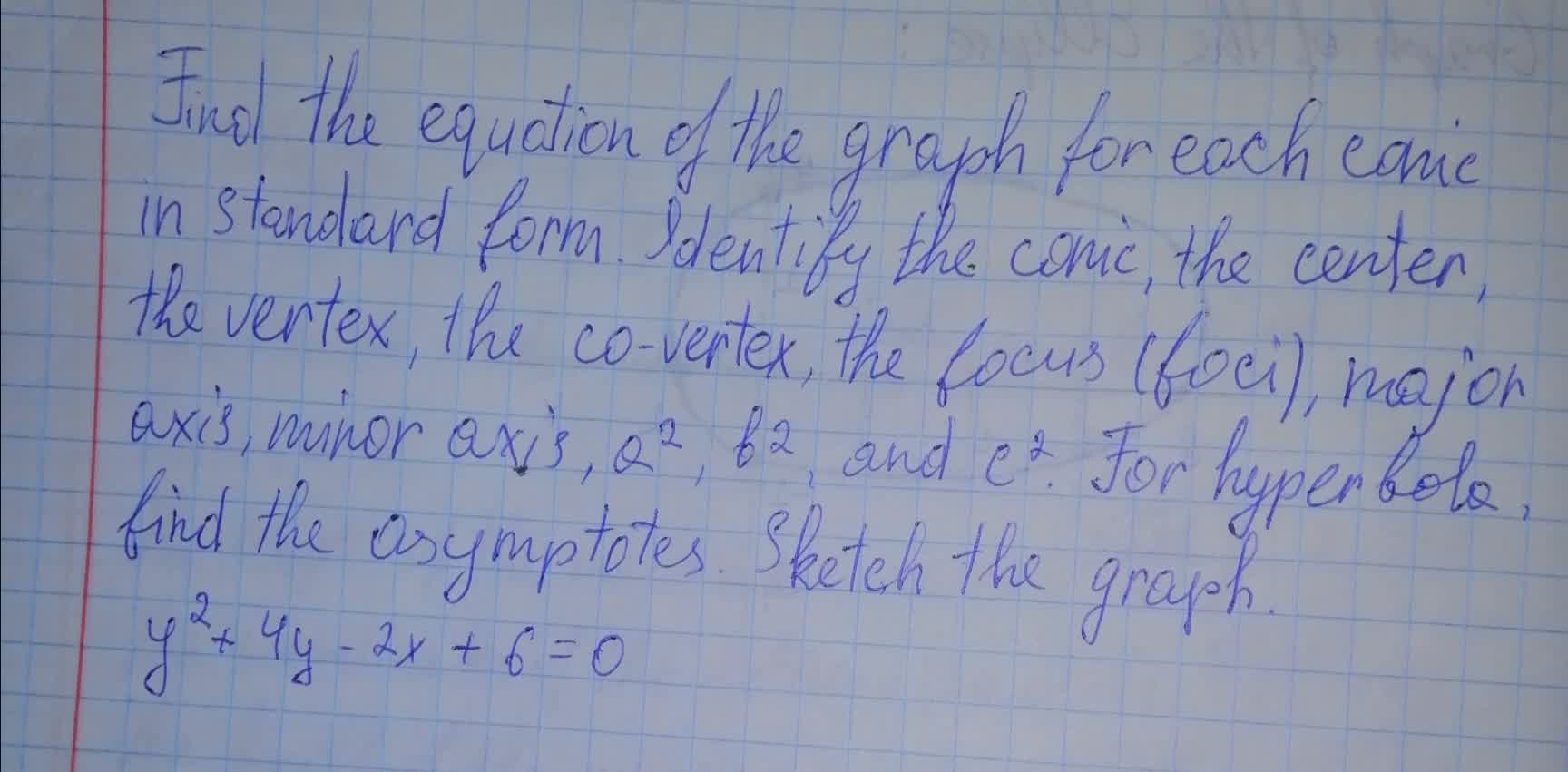floymdiT 2021-08-09 Answered

### Find the equation of the graph for each conic in general form. Identify the conic, the center, the vertex, the co-vertex, the focus (foci), major axis, minor axis, $$\displaystyle{a}^{{2}}$$, $$\displaystyle{b}^{{2}}$$, and $$\displaystyle{c}^{{2}}$$. For hyperbola,find the asymtotes.Sketch the graph $$\displaystyle{\left({x}-{2}\right)}^{{2}}+{\left({y}-{1}\right)}^{{2}}={49}$$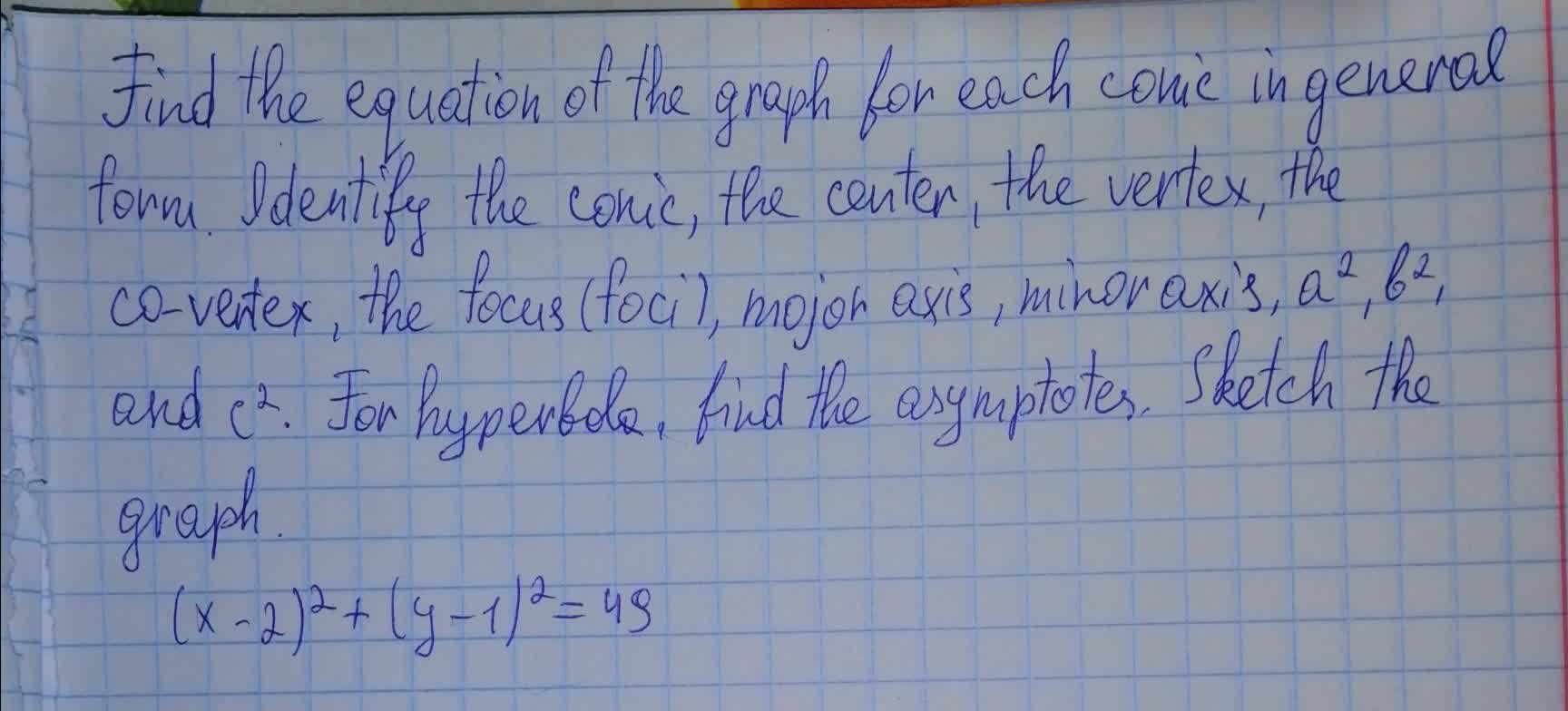Elleanor Mckenzie 2021-08-08 Answered

### A solid is formed by cutting a conical section away from a right circular cylinder. If the radius measures 6 in. and the altitude measures 8 in., what is the volume of the resulting solid?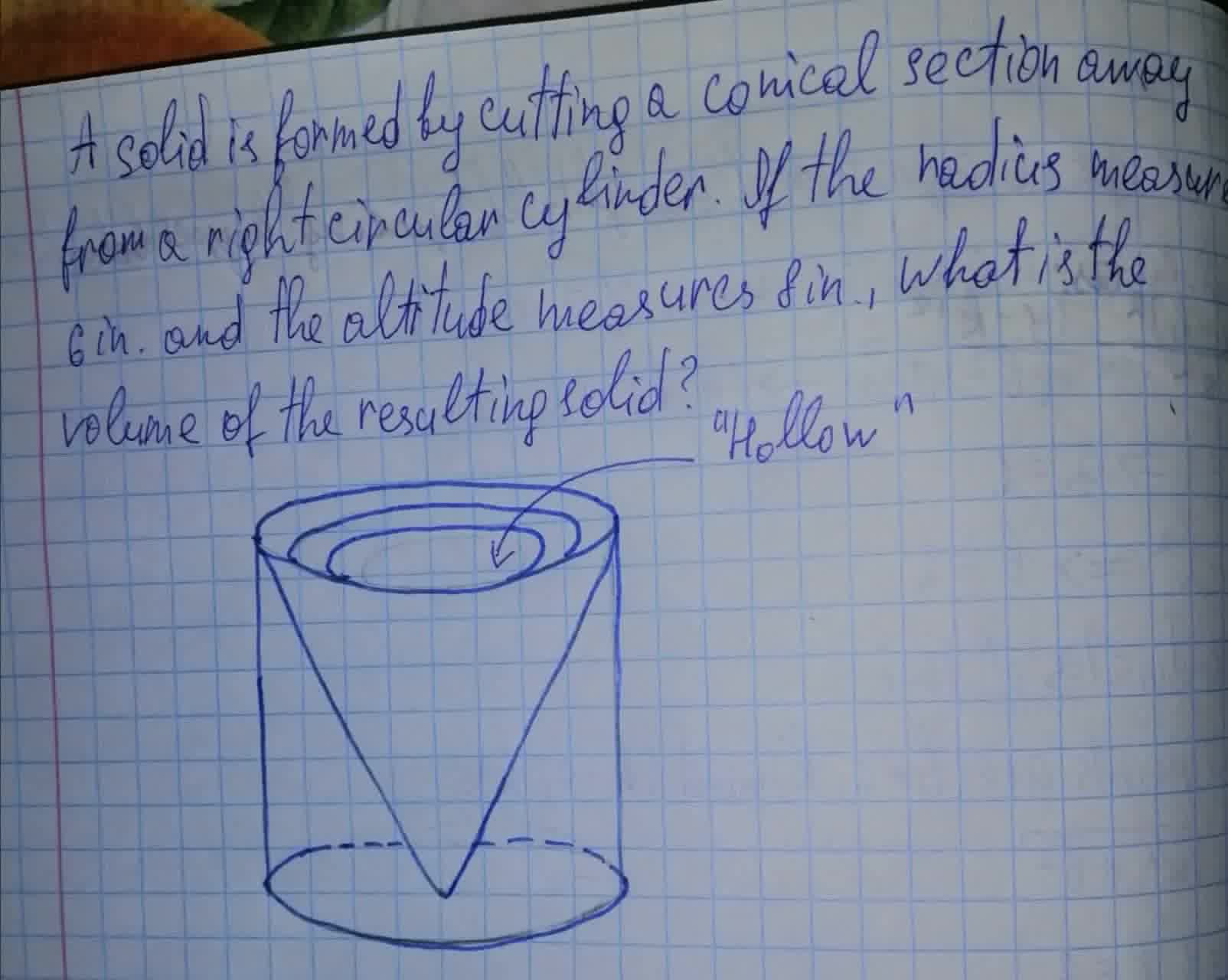Jason Farmer 2021-08-08 Answered

### A polar equation of a conic is given. $$\displaystyle{r}=\frac{{8}}{{1}}+{2}{\cos{{\left(\theta\right)}}}$$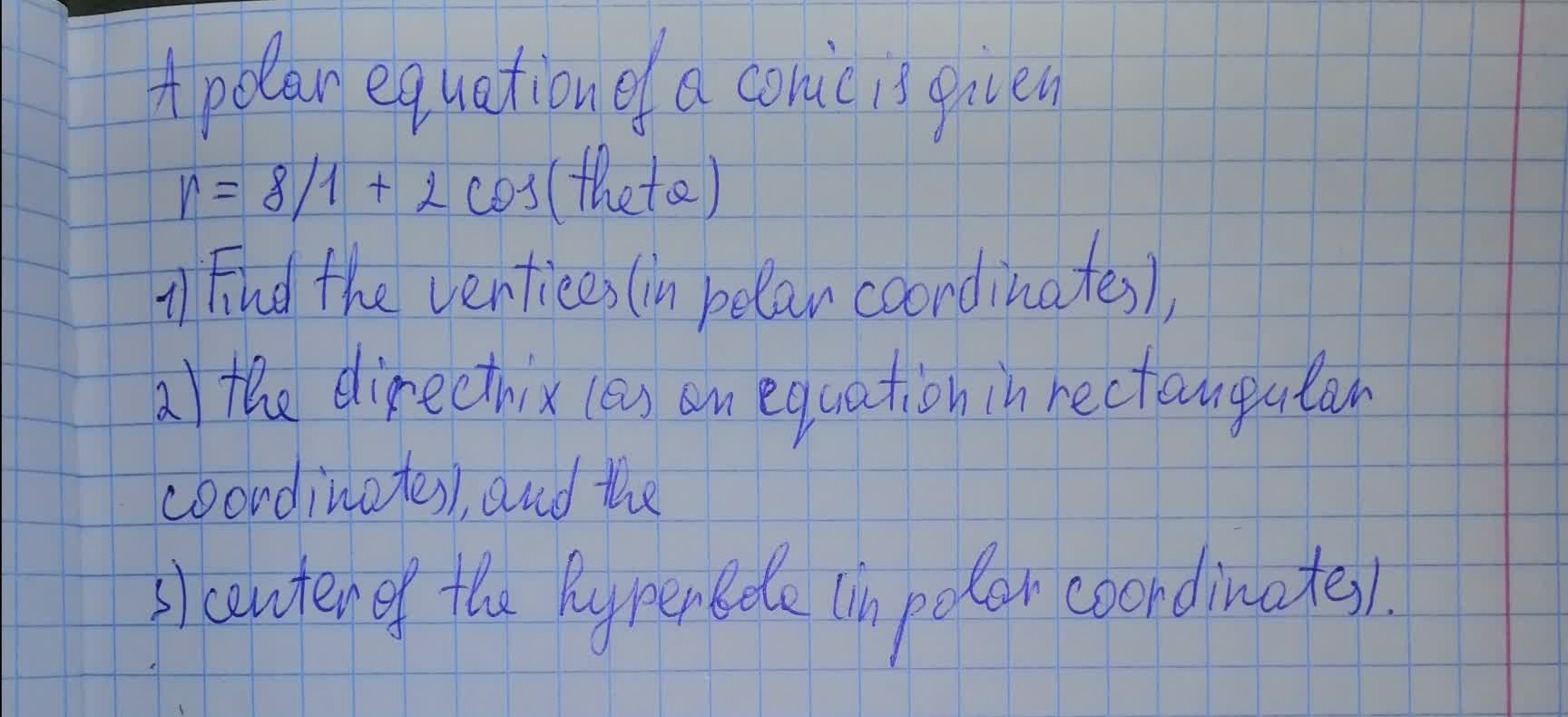BolkowN 2021-08-08 Answered

### Find an equation of the conic described.Graph the equation. Parabola; vertex at (0,0); directrix the line y=-3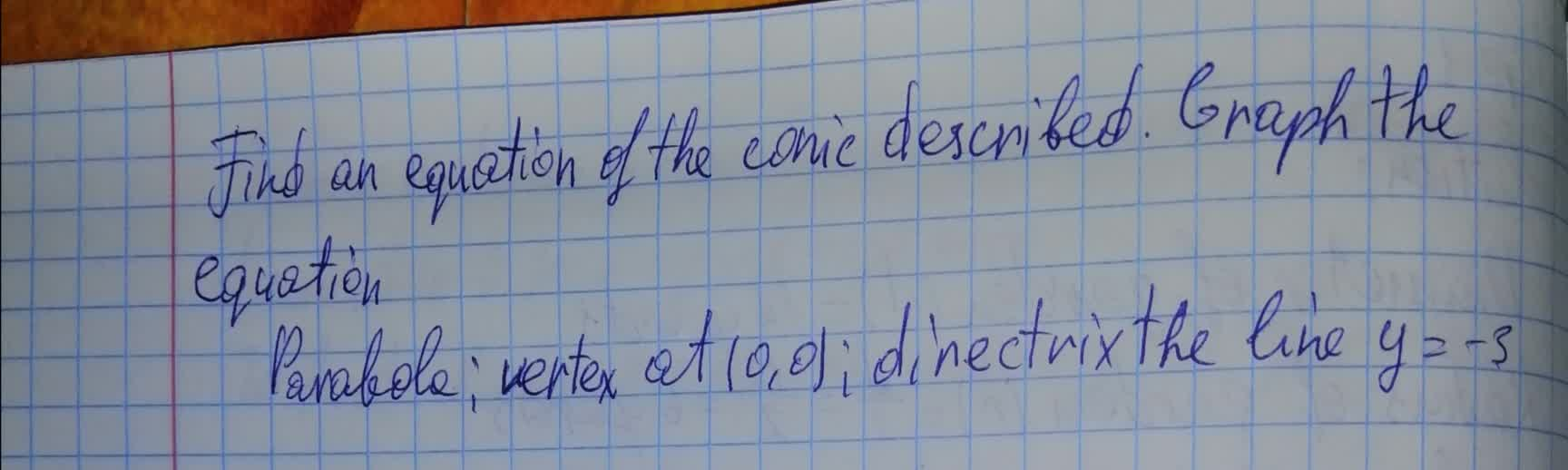Jaya Legge 2021-08-08 Answered

### Find the equation by determining the type of the conic and draw its graph. $$\displaystyle{25}{x}^{{2}}+{9}{y}^{{2}}-{100}{x}+{54}{y}-{44}={0}$$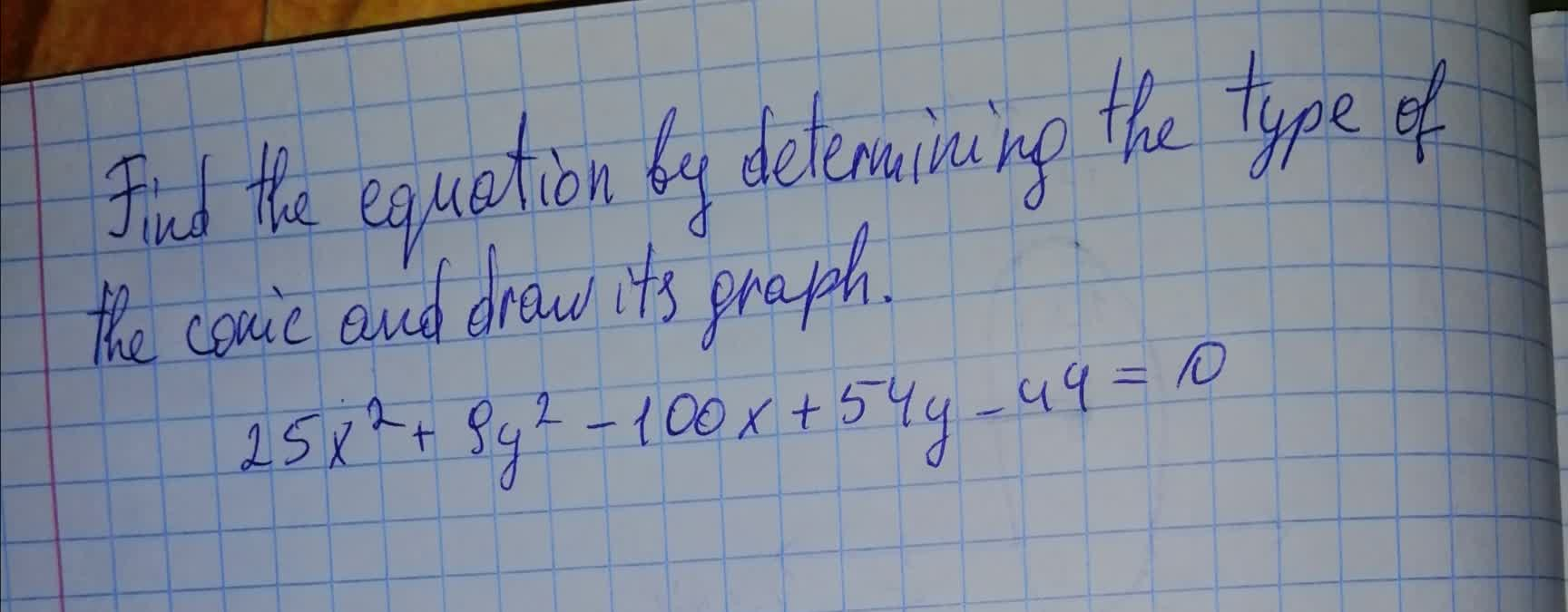lwfrgin 2021-08-08 Answered

### Find the equations of the parabolas that share a vertex and a focus with the ellipse. Draw the conics. $$\displaystyle{25}{x}^{{2}}+{9}{y}^{{2}}={9}$$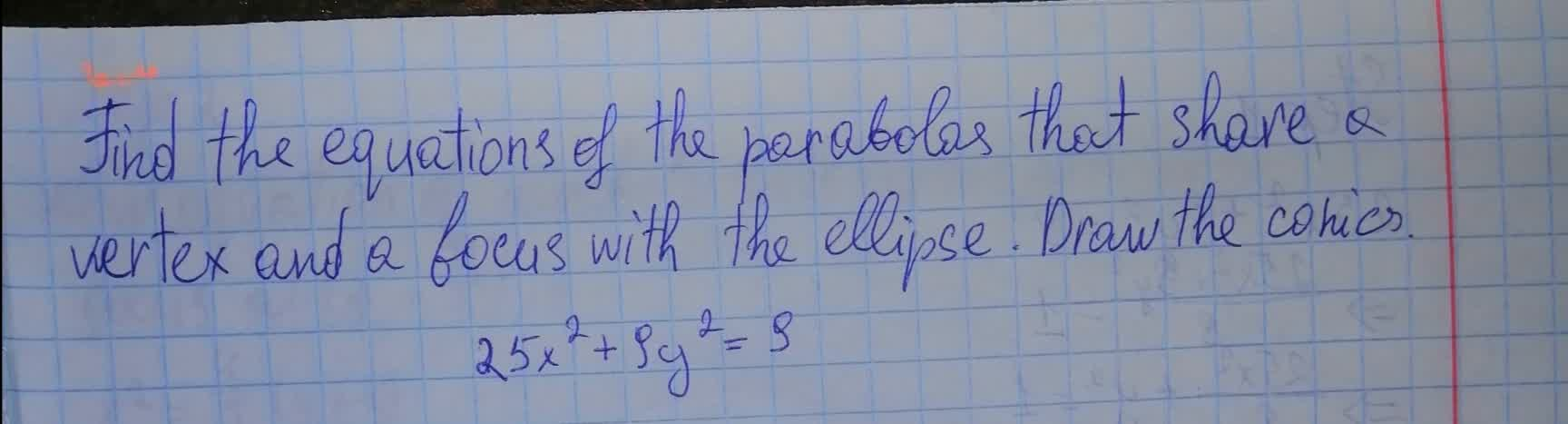Trent Carpenter 2021-08-08 Answered

### Find the equation of the graph for each conic in general form. Identify the conic, the center, the vertex, the co-vertex, the focus (foci), major axis, minor axis, $$\displaystyle{a}^{{2}}$$, $$\displaystyle{b}^{{2}}$$, and $$\displaystyle{c}^{{2}}$$. For hyperbola,find the asymtotes.Sketch the graph $$\displaystyle{\left({y}+{7}\right)}^{{2}}+{\left({x}-{5}\right)}^{{2}}={25}$$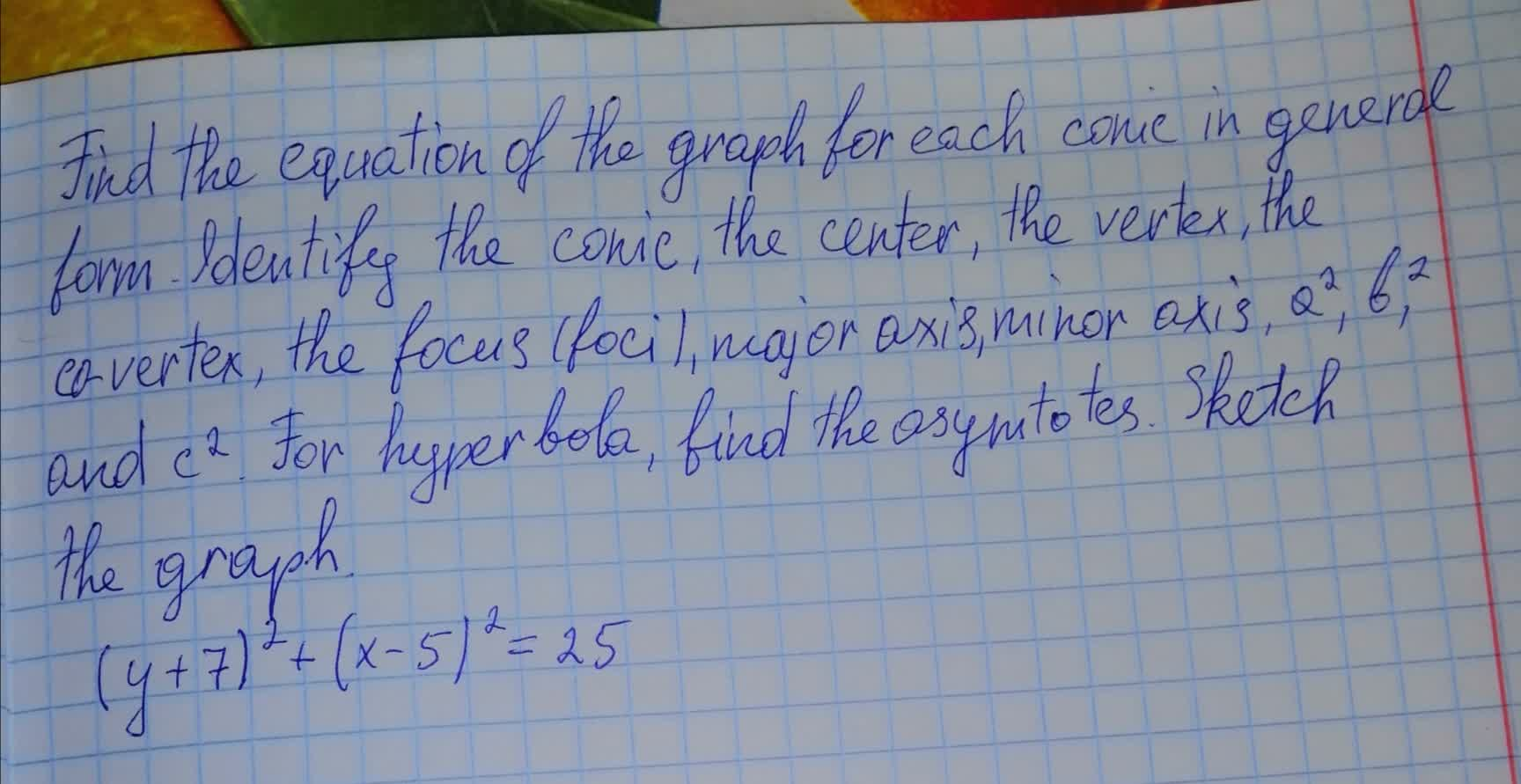texelaare 2021-08-08 Answered

### Find the equation of the graph for each conic in general form. Identify the conic, the center, the vertex, the co-vertex, the focus (foci), major axis, minor axis, $$\displaystyle{a}^{{2}}$$, $$\displaystyle{b}^{{2}}$$, and $$\displaystyle{c}^{{2}}$$. For hyperbola,find the asymtotes.Sketch the graph $$\displaystyle{9}{\left({y}-{3}\right)}^{{2}}-{4}{\left({x}+{5}\right)}^{{2}}={36}$$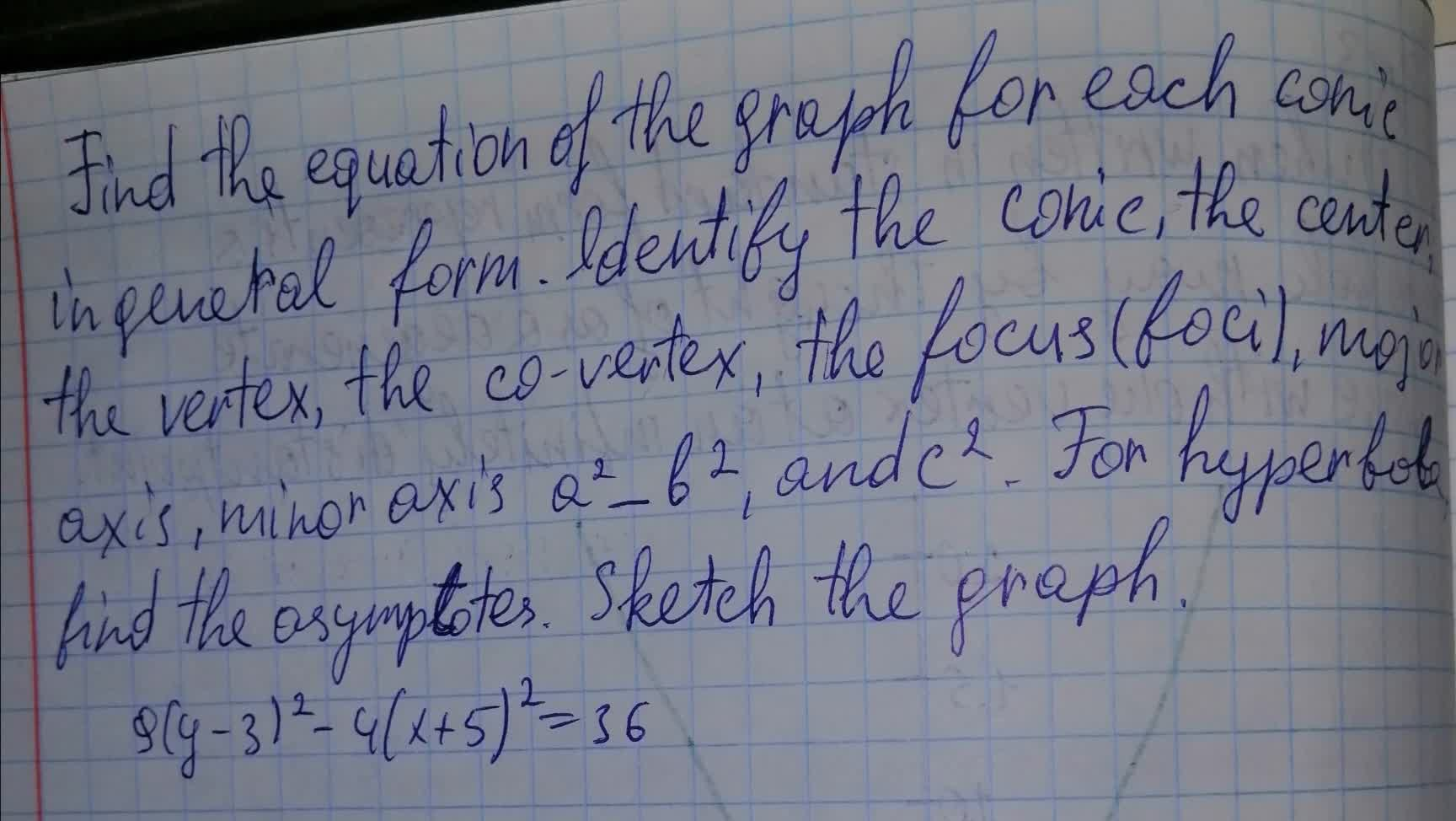ringearV 2021-08-08 Answered

### Find the largest and smallest distances from the origin to the conic whose equation is $$\displaystyle{5}{x}^{{2}}-{6}{x}{y}+{5}{y}^{{2}}-{32}={0}$$ and hence determine the lengths of the semiaxes of this conic.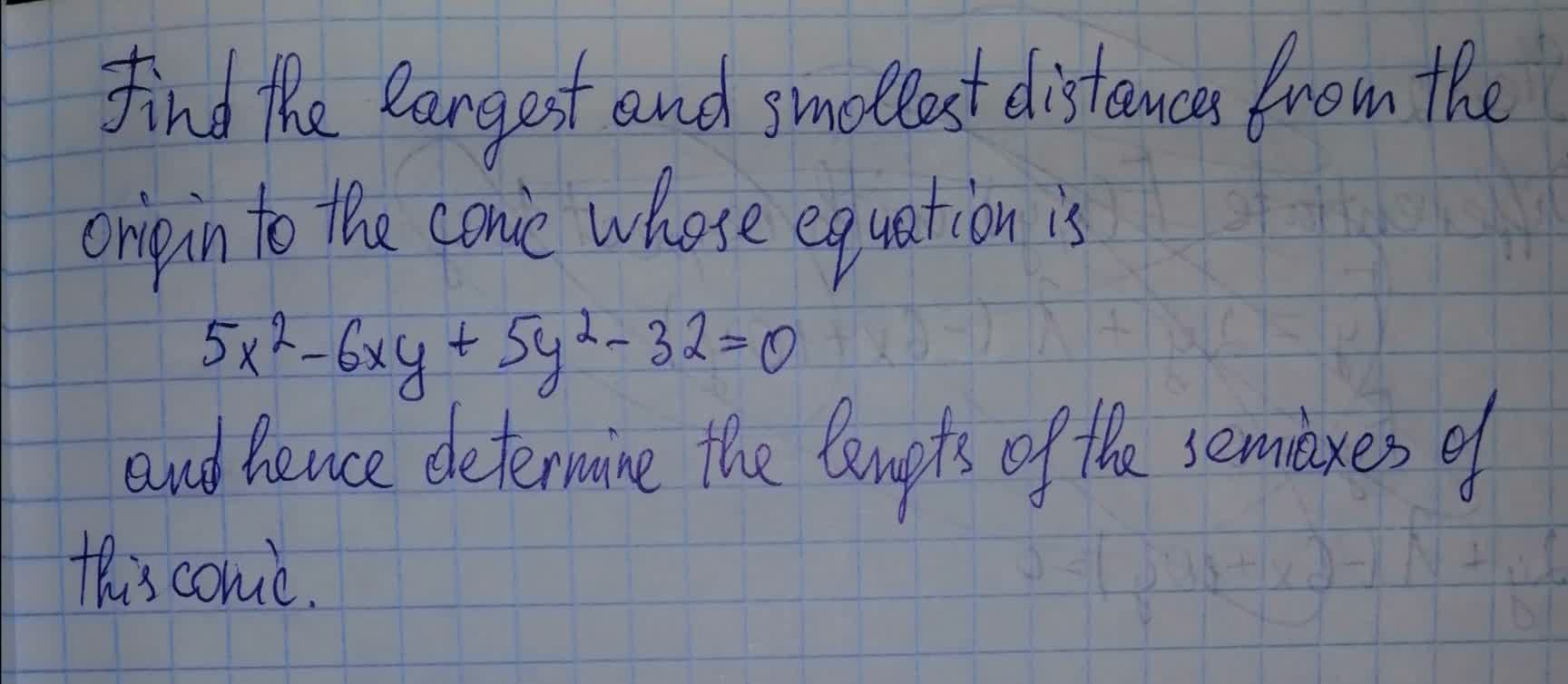usagirl007A 2021-08-08 Answered

### Draw the curve of the given equations a. $$\displaystyle{x}^{{2}}+{y}^{{2}}+{6}{x}-{12}{y}+{29}={0}$$, b. $$\displaystyle{y}^{{2}}-{w}{x}-{8}{y}+{10}={0}$$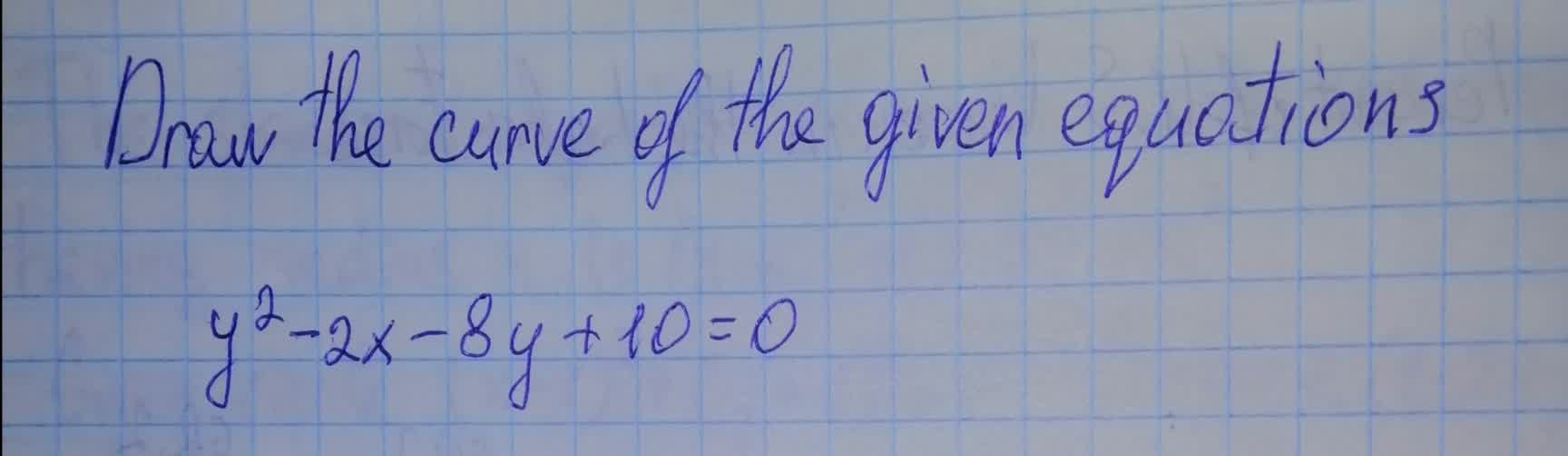Mylo O'Moore 2021-08-07 Answered

### Find the equation of the graph for each conic in general form. Identify the conic , the center , the vertex , the co-vertex , the focus(foci) , major axis , minor axis , $$\displaystyle{a}^{{2}}$$ , $$\displaystyle{b}^{{2}}$$ and $$\displaystyle{c}^{{2}}$$. For hyperbola , find the asymptotes. Sketch the graph. $$\displaystyle{25}{\left({x}+{2}\right)}^{{2}}-{144}{\left({y}-{1}\right)}^{{2}}={\left({25}\right)}{\left({144}\right)}$$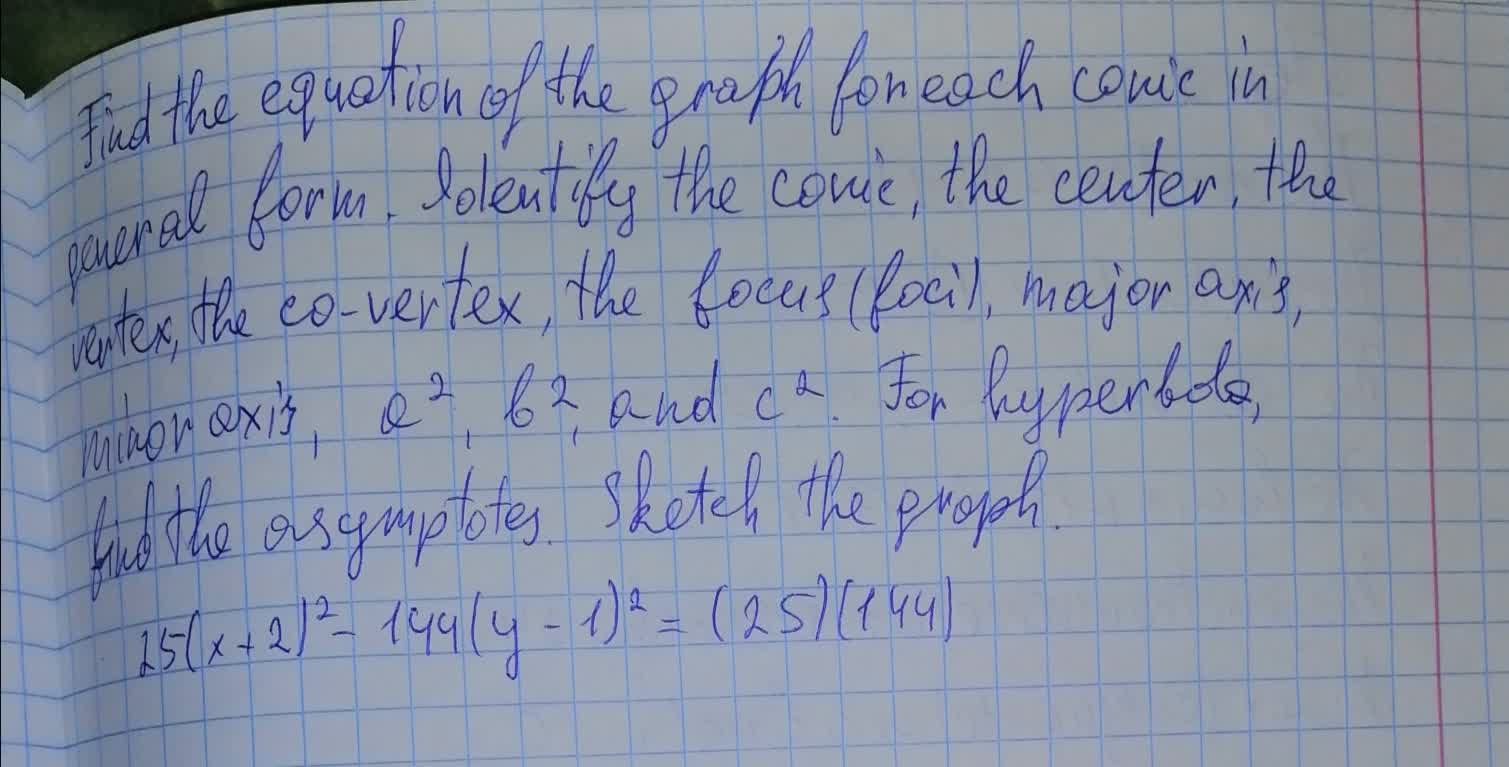a2linetagadaW 2021-08-07 Answered

### Identify and sketch the graph of the conic section. $$\displaystyle{9}{x}^{{2}}+{9}{y}^{{2}}+{18}{x}-{18}{y}+{14}={0}$$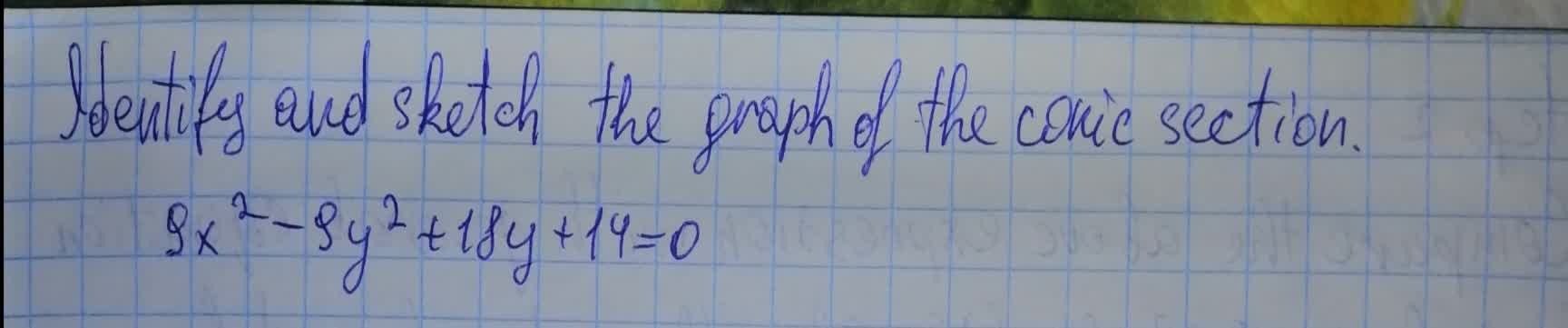vestirme4 2021-08-07 Answered

### Find the equation of the graph for each conic in standard form. Identify the conic, the center, the vertex, the co-vertex, the focus (foci), major axis, minor axis, $$\displaystyle{a}^{{2}},{b}^{{2}}$$, and $$\displaystyle{c}^{{2}}$$?. For hyperbola, find the asymptotes. Sketch the graph. $$\displaystyle{9}{x}^{{2}}-{y}^{{2}}+{36}{x}+{4}{y}+{23}={0}$$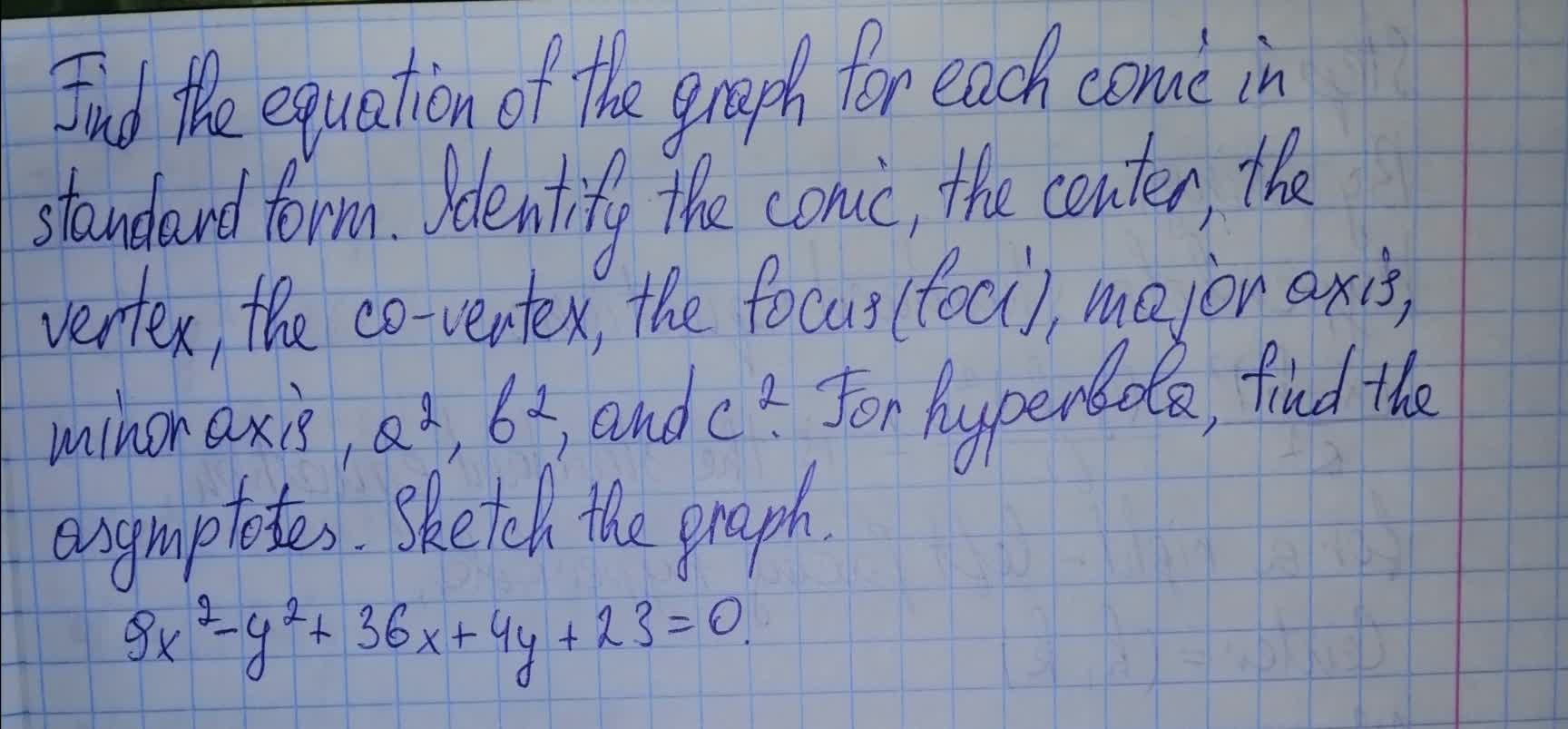tinfoQ 2021-08-07 Answered

...